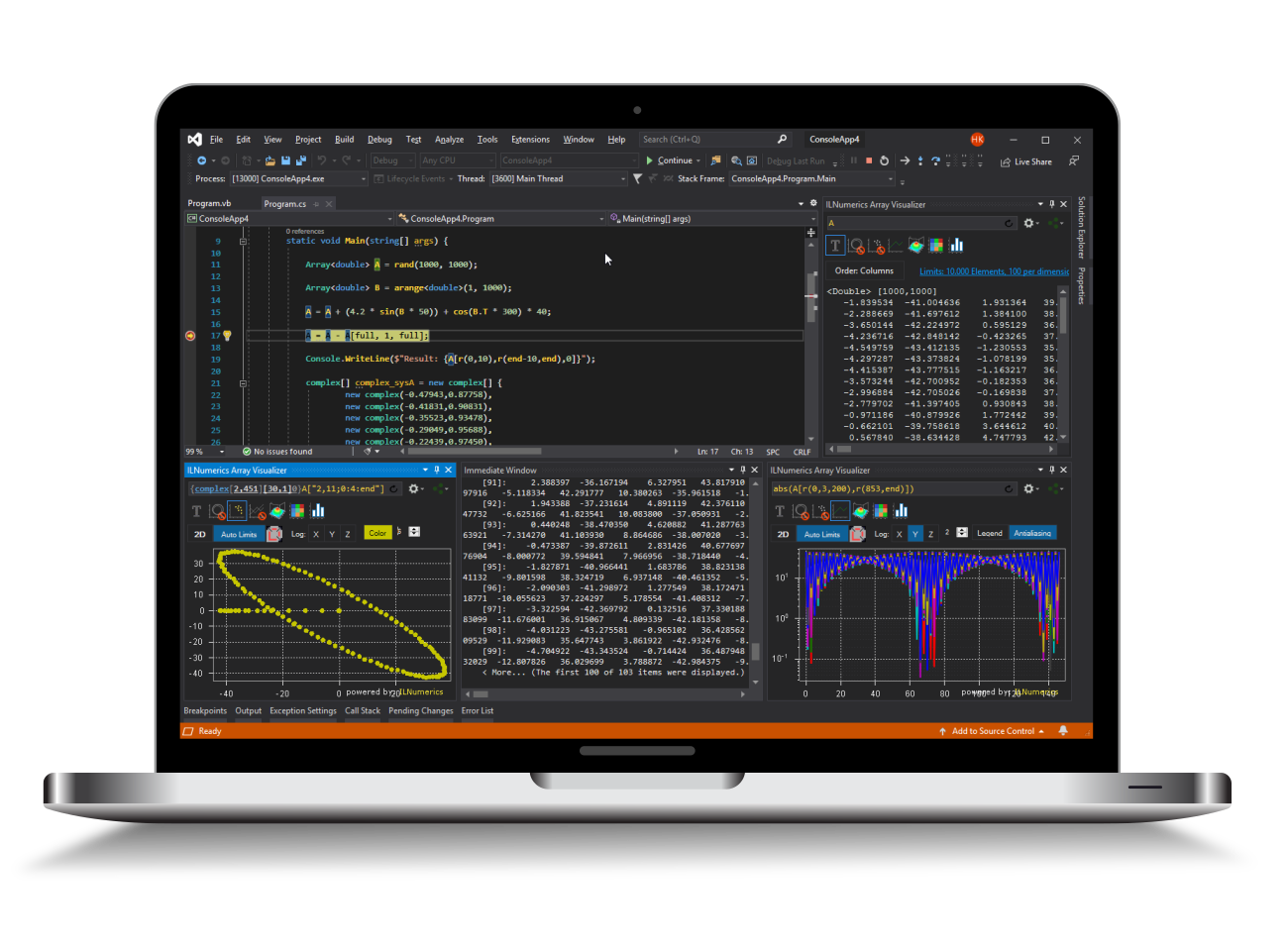Industrial Data Science
in C# and .NET:
Simple. Fast. Reliable.ILNumerics - Technical Computing

Modern High Performance Tools for Technical

Computing and Visualization in Industry and Science

tgt

# Contour Plots

From Wikipedia: “… a contour line for a function of two variables is a curve connecting points where the function has the same particular value. The gradient of the function is always perpendicular to the contour lines. When the lines are close together the magnitude of the gradient is large: the variation is steep.”

Contour plots create contour lines connecting neighboring points of equal value in a matrix of data. The matrix may describe the heights of some points in 3D space. For landmarks X and Y coordinates might specify the position of the points, Z might be some arbitrary value like the height, temperature, pressure, population, pollution and so on. The contour lines make it easier to locate regions with similar properties and to identify clusters within the data.

The following contour plot creates contour lines for equal heights in a real world height map data set included in ILNumerics: